# 10-year Inflation-Indexed Bonds (JGBi)

10-year Inflation-Indexed Bonds (JGBi), introduced in March 2004, are the government bonds of which principal amount fluctuates in proportion with the consumer price index―i.e. CPI excluding fresh food. While this new instrument meets the needs of investors who want to avert inflationary risks, it can also serve as a means of observing the expected inflation rate in the market.
With conventional fixed-rate coupon-bearing JGBs, the principal at the time of issuance remains unchanged till redemption, and the interest amount remains the same for biannual interest payment. On the contrary, with JGBi, the principal amount fluctuates in proportion with the CPI. Therefore, if the CPI increases after issue, the principal amount also increases according to the rate of inflation, and vice versa, and at maturity they are redeemed at the adjusted principal amount (hereinafter called “inflation-adjusted principal amount”). The principal amount of JGBi issued in and after 2013 will be guaranteed at maturity (deflation floor). In case where the indexation coefficient falls below 1 at maturity, the Bonds will be redeemed at the face value.
Interest amount also changes according to the rate of inflation as it is calculated by multiplying the inflation-adjusted principal amount at the time of interest payment by the pre-fixed coupon rate.
Inflation-adjusted principal amount is calculated by multiplying face value by indexation coefficient. Indexation coefficient, which indicates the level of fluctuation from the time of issuance, is calculated by dividing Ref index for the day by Ref index at the time of issuance (specifically, the 10th day of the issue month).

[Conceptual scheme of Inflation-Indexed Bonds]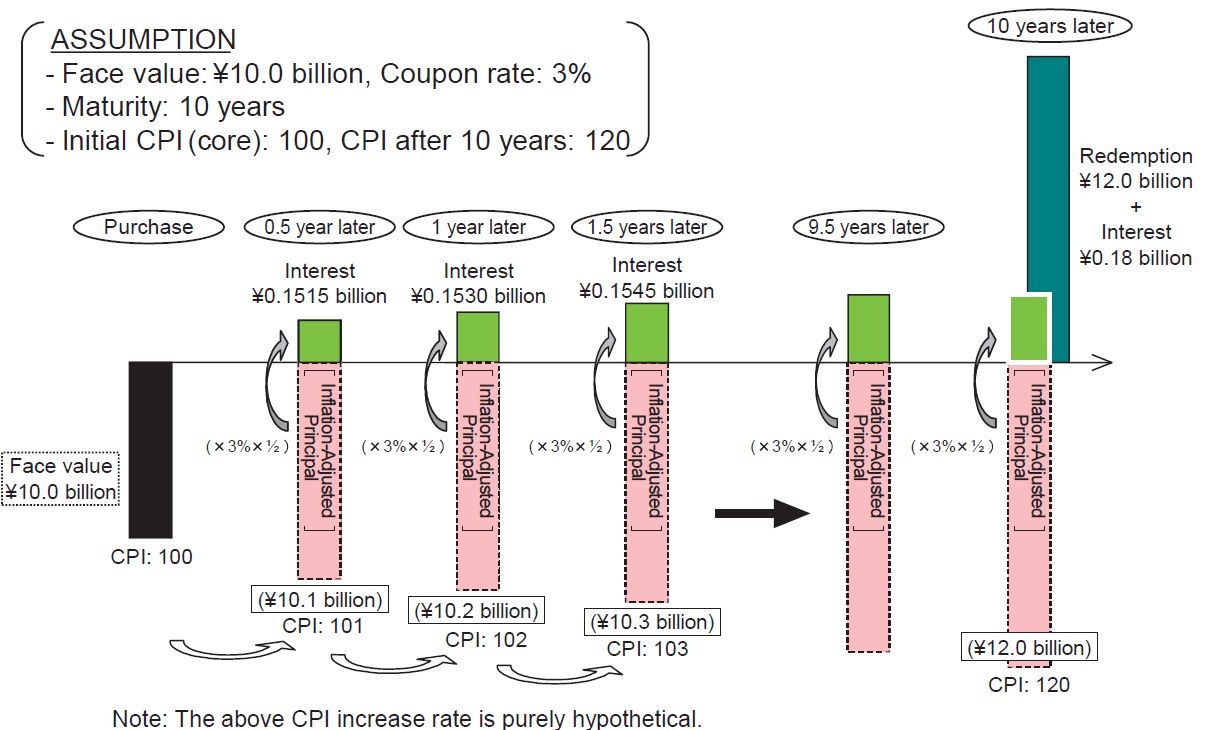[Deflation Floor(Conceptual Diagram)]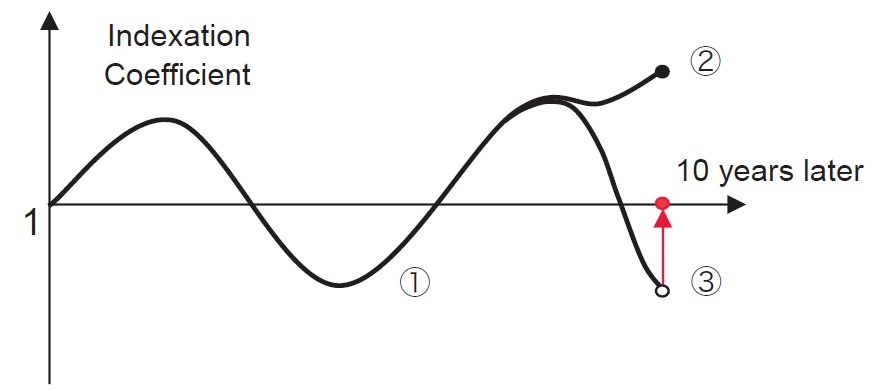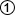In case where the indexation coefficient is below 1, theinflation-adjusted principal will fall below its face value.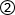In case where the indexation coefficient at maturity is above 1,the principal and the interest will be paid based on the     inflation-adjusted principal per se.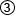In case where the indexation coefficient at maturity is below 1, principal will be redeemed at its face value.
Note:Deflation floor has no effect on interests during the maturing period or at maturity.